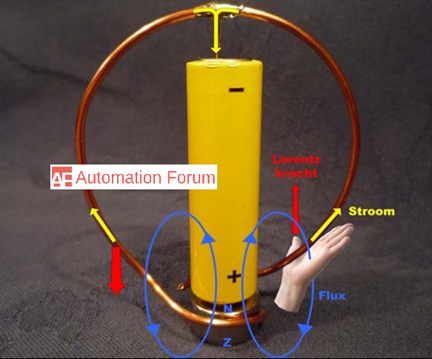# What is a homoplar motor and how does it work? How to construct a homopolar motor

What is an electric motor and for what purpose it is used

Electric motors are devices that can transform electrical energy into mechanical energy by means of electromagnetic fields. There are some motors that can do the reverse, they can transform mechanical energy into electrical energy to operate as generators. The two major part of an electrical motor is stator and rotor, the stator is the fixed part and the rotor is the mobile part. Stator would act as a base that allows performing the rotation of the motor, and the rotation is not done mechanically it is done magnetically and the rotor is the rotating element that allows the transmission of mechanical energy.

What is a homopolar motor

A homopolar motor is a direct current electric motor, which has two magnetic poles providing a static magnetic field. The homopolar electric generation process is done by using a moving electric conductor and this conductor will be enclosed by a unidirectional and constant magnetic field. In this process, there is a strict relationship between the electric field, magnetic field, and inertia. The electric power which is generated is determined by their magnitude. If there is a flowing electric current then there will be a magnetic field too and reverse will happen too. Inertia is formed because of the moving mass in the surrounding space. The conductor will always cut unidirectional lines of magnetic flux by rotating the conductor around a fixed axis which is parallel to the magnetic field. The produced EMF will be continuous in one direction, the homopolar motor doesn’t need a commuter but it would need slip rings. The name homopolar indicates that the electrical polarity of the conductor and the magnetic field poles do not change.

The operating principle of a homopolar motor is by the Lorentz force. When the current flows through a conductor that is placed in a magnetic field that is perpendicular to the current, then it will experience a force in a direction perpendicular to both the magnetic field and current. The electromagnetic force can be used to provide a torque around the axis of rotation. There is no need for commuter because the direction of current and magnetic field poles does not change. A homopolar motor is considered to be the first electric motor to be built.

How does the homopolar motor workIn the homopolar motor the electric conductor, which is movable bounded to a direction and it will remain in the constant magnetic field. In principle, there is a relation between the magnetic field, electric current and moment of inertia. Their magnitudes are calculated by the generated electric power. There will be a constant magnetic field in the current-carrying wire and that’s why the wire rotates. When the conductor moves through the magnetic field it would be opposed by a force and will be pushed through the magnetic field and this force will create a torque around the axis of rotation. It’s because the axis of rotation is parallel to the magnetic field, and the opposing magnetic fields do not change polarity, so no commutation is needed to turn the conductor, and it is achieved by the help of single-coil turn, but it would make this homopolar motor unsuited for many applications.

Because of the Lorentz force in the winding, the motor will start rotating. The winding is an electrical current conductor through which the current of the battery will flow and this current will induce a magnetic field around the wire. The neodymium magnet also induces a strong magnetic field and these two magnetic fields are repulsive, which would cause the Lorentz force and rotation.

How can we use the homopolar motor as a generator

A homopolar motor is reversible like most of the electromechanical machines, if the conductor is turned mechanically then it will act as a homopolar generator. Between the two terminals of the motor, a direct current voltage will be produced because of this.

How to construct a homopolar motor and what do we need to construct it

The construction of a homopolar motor is very simple, a permanent magnet is used for the external magnetic field in which the conductor will rotate. The battery is used so that the current could flow along the conducting wire. There is no need to move the magnet or to be in contact with the rest of the motor. Its only purpose is to provide a magnetic field, which will interact with the magnetic field induced by the current in the wire. A magnet can be attached to the battery and allow the conducting wire to rotate freely.

Efficiency of homopolar motor

The homopolar motor will work efficiently if there is better contact with the magnet and wire. The wire will heat after some time, changing the polarity of the magnet or of the stack will change the rotation of the wire. Homopolar motor speed can be modified by changing the battery or magnet, or by varying the wire gauge.